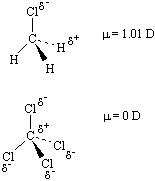The Covalent Bond

Valence Electrons

The electrons in the outermost shell are the valence electronsthe electrons on an atom that can be gained or lost in a chemical reaction. Since filled d or f subshells are seldom disturbed in a chemical reaction, we can define valence electrons as follows: The electrons on an atom that are not present in the previous rare gas, ignoring filled d or f subshells.

Gallium has the following electron configuration.

Ga: [Ar] 4s2 3d10 4p1

The 4s and 4p electrons can be lost in a chemical reaction, but not the electrons in the filled 3d subshell. Gallium therefore has three valence electrons.

 Practice Problem 1:Determine the number of valence electrons in neutral atoms of the following elements: (a) Si (b) Mn (c) Sb (d) Pb Click here to check your answer to Practice Problem 1The Covalent Bond

Atoms can combine to achieve an octet of valence electrons by sharing electrons. Two fluorine atoms, for example, can form a stable F2 molecule in which each atom has an octet of valence electrons by sharing a pair of electrons.A pair of oxygen atoms can form an O2 molecule in which each atom has a total of eight valence electrons by sharing two pairs of electrons.

The term covalent bond is used to describe the bonds in compounds that result from the sharing of one or more pairs of electrons.How Sharing of Electrons Bonds Atoms

To understand how sharing a pair of electrons can hold atoms together, let's look at the simplest covalent bondthe bond that forms when two isolated hydrogen atoms come together to form an H2 molecule.

H · + · HH-H

An isolated hydrogen atom contains one proton and one electron held together by the force of attraction between oppositely charged particles. The magnitude of this force is equal to the product of the charge on the electron (qe) times the charge on the proton (qp) divided by the square of the distance between these particles (r2).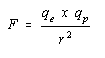When a pair of isolated hydrogen atoms are brought together, two new forces of attraction appear because of the attraction between the electron on one atom and the proton on the other.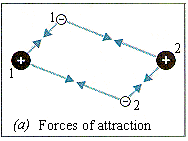But two forces of repulsion are also created because the two negatively charged electrons repel each other, as do the two positively charged protons.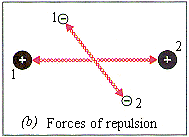It might seem that the two new repulsive forces would balance the two new attractive forces. If this happened, the H2 molecule would be no more stable than a pair of isolated hydrogen atoms. But there are ways in which the forces of repulsion can be minimized. As we have seen, electrons behave as if they were tops spinning on an axis. Just as there are two ways in which a top can spin, there are two possible states for the spin of an electron: s = +1/2 and s = -1/2. When electrons are paired so that they have opposite spins, the force of repulsion between these electrons is minimized.

The force of repulsion between the protons can be minimized by placing the pair of electrons between the two nuclei. The distance between the electron on one atom and the nucleus of the other is now smaller than the distance between the two nuclei. As a result, the force of attraction between each electron and the nucleus of the other atom is larger than the force of repulsion between the two nuclei, as long as the nuclei are not brought too close together.

The net result of pairing the electrons and placing them between the two nuclei is a system that is more stable than a pair of isolated atoms if the nuclei are close enough together to share the pair of electrons, but not so close that repulsion between the nuclei becomes too large. The hydrogen atoms in an H2 molecule are therefore held together (or bonded) by the sharing of a pair of electrons and this bond is the strongest when the distance between the two nuclei is about 0.074 nm.Similarities and Differences Between Ionic and Covalent Compounds

There is a significant difference between the physical properties of NaCl and Cl2, as shown in the table below, which results from the difference between the ionic bonds in NaCl and the covalent bonds in Cl2.

Some Physical Properties of NaCl and Cl2

 NaCl Cl2 Phase at room temperature Solid Gas Density 2.165 g/cm3 0.003214 g/cm3 Melting point 801°C -100.98°C Boiling point 1413°C -34.6°C Ability of aqueous solution to conduct electricity Conducts Does not conduct

Each Na+ ion in NaCl is surrounded by six Cl- ions, and vice versa, as shown in the figure below. Removing an ion from this compound therefore involves breaking at least six bonds. Some of these bonds would have to be broken to melt NaCl, and they would all have to be broken to boil this compound. As a result, ionic compounds such as NaCl tend to have high melting points and boiling points. Ionic compounds are therefore solids at room temperature.Cl2 consists of molecules in which one atom is tightly bound to another, as shown in the figure above. The covalent bonds within these molecules are at least as strong as an ionic bond, but we don't have to break these covalent bonds to separate one Cl2 molecule from another. As a result, it is much easier to melt Cl2 to form a liquid or boil it to form a gas, and Cl2 is a gas at room temperature.

The difference between ionic and covalent bonds also explains why aqueous solutions of ionic compounds conduct electricity, while aqueous solutions of covalent compounds do not. When a salt dissolves in water, the ions are released into solution.

 H2O NaCl(s)Na+(aq) + Cl-(aq)

These ions can flow through the solution, producing an electric current that completes the circuit. When a covalent compound dissolves in water, neutral molecules are released into the solution, which cannot carry an electric current.

 H2O C12H22O11(s)C12H22O11(aq)

When two chlorine atoms come together to form a covalent bond, each atom contributes one electron to form a pair of electrons shared equally by the two atoms, as shown in the figure below. When a sodium atom combines with a chlorine atom to form an ionic bond, each atom still contributes one electron to form a pair of electrons, but this pair of electrons is not shared by the two atoms. The electrons spend most of their time on the chlorine atom.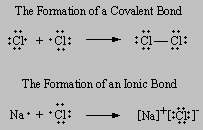Ionic and covalent bonds differ in the extent to which a pair of electrons is shared by the atoms that form the bond. When one of the atoms is much better at drawing electrons toward itself than the other, the bond is ionic. When the atoms are approximately equal in their ability to draw electrons toward themselves, the atoms share the pair of electrons more or less equally, and the bond is covalent. As a rule of thumb, metals often react with nonmetals to form ionic compounds or salts, and nonmetals combine with other nonmetals to form covalent compounds. This rule of thumb is useful, but it is also naive, for two reasons.

• The only way to tell whether a compound is ionic or covalent is to measure the relative ability of the atoms to draw electrons in a bond toward themselves.
• Any attempt to divide compounds into just two classes (ionic and covalent) is doomed to failure because the bonding in many compounds falls between these two extremes.

The first limitation is the basis of the concept of electronegativity. The second serves as the basis for the concept of polarity.Electronegativity

The relative ability of an atom to draw electrons in a bond toward itself is called the electronegativity of the atom. Atoms with large electronegativities (such as F and O) attract the electrons in a bond better than those that have small electronegativities (such as Na and Mg). The electronegativities of the main group elements are given in the figure below.When the magnitude of the electronegativities of the main group elements is added to the periodic table as a third axis, we get the results shown in the figure below.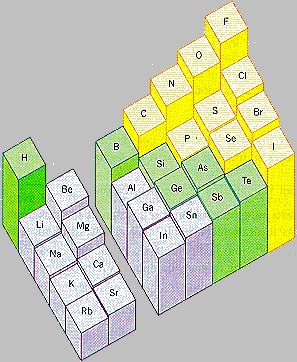There are several clear patterns in the data in the above two figures.

• Electronegativity increases in a regular fashion from left to right across a row of the periodic table.
• Electronegativity decreases down a column of the periodic table.Using Electronegativity to Identify Ionic, Covalent, and Polar Covalent Compounds

When the difference between the electronegativities of the elements in a compound is relatively large, the compound is best classified as ionic.

Example: NaCl, LiF, and SrBr2 are good examples of ionic compounds. In each case, the electronegativity of the nonmetal is at least two units larger than that of the metal.

 NaCl LiF SrBr2 Cl EN = 3.16 F EN = 3.98 Br EN = 2.96 Na EN = 0.93 Li EN = 0.98 Sr EN = 0.95 ¯¯¯¯¯¯¯¯¯ ¯¯¯¯¯¯¯¯¯ ¯¯¯¯¯¯¯¯¯EN = 2.23EN = 3.00EN = 2.01

We can therefore assume a net transfer of electrons from the metal to the nonmetal to form positive and negative ions and write the Lewis structures of these compounds as shown in in the figure below.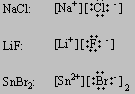These compounds all have high melting points and boiling points, as might be expected for ionic compounds.

 NaCl LiF SrBr2 MP 801oC 846oC 657oC BP 1413oC 1717oC 2146oC

They also dissolve in water to give aqueous solutions that conduct electricity, as would be expected.

When the electronegativities of the elements in a compound are about the same, the atoms share electrons, and the substance is covalent.

Example: Examples of of covalent compounds include methane (CH4), nitrogen dioxide (NO2), and sulfur dioxide (SO2).

 CH4 NO2 SO2 C EN = 2.55 O EN = 3.44 O EN = 3.44 H EN = 2.20 N EN = 3.04 S EN = 2.58 ¯¯¯¯¯¯¯¯¯ ¯¯¯¯¯¯¯¯¯ ¯¯¯¯¯¯¯¯¯EN = 0.35EN = 0.40EN = 0.86

These compounds have relatively low melting points and boiling points, as might be expected for covalent compounds, and they are all gases at room temperature.

 CH4 NO2 SO2 MP -182.5oC -163.6oC -75.5oC BP -161.5oC -151.8oC -10oC

Inevitably, there must be compounds that fall between these extremes. For these compounds, the difference between the electronegativities of the elements is large enough to be significant, but not large enough to classify the compound as ionic. Consider water, for example.

 H2O O EN = 3.44 H EN = 2.20 ¯¯¯¯¯¯¯¯¯EN = 1.24

Water is neither purely ionic nor purely covalent. It doesn't contain positive and negative ions, as indicated by the Lewis structure on the left in the figure below. But the electrons are not shared equally, as indicated by the Lewis structure on the right in this figure. Water is best described as a polar compound. One end, or pole, of the molecule has a partial positive charge (+), and the other end has a partial negative charge (-).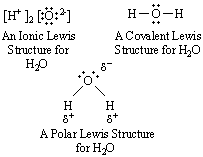As a rule, when the difference between the electronegativities of two elements is less than 1.2, we assume that the bond between atoms of these elements is covalent. When the difference is larger than 1.8, the bond is assumed to be ionic. Compounds for which the electronegativity difference is between about 1.2 and 1.8 are best described as polar, or polar covalent.

 Covalent:EN < 1.2 Polar: 1.2 <EN < 1.8 Ionic:EN > 1.8

 Practice Problem 2:Use electronegativities to decide whether the following compounds are best described as either covalent, ionic, or polar. (a) Sodium cyanide (NaCN) (b) Tetraphosphorus decasulfide (P4S10) (c) Carbon monoxide (CO) (d) Silicon tetrachloride (SiCl4) Click here to check your answer to Practice Problem 2Limitations of the Electronegativity Concept

Electronegativity summarizes the tendency of an element to gain, lose, or share electrons when it combines with another element. But there are limits to the success with which it can be applied. BF3 (EN = 1.94) and SiF4 (EN = 2.08), for example, have electronegativity differences that lead us to expect these compounds to behave as if they were ionic, but both compounds are covalent. They are both gases at room temperature, and their boiling points are -99.9oC and -86oC, respectively.

The source of this problem is that each element is assigned only one electronegativity value, which is used for all of its compounds. But fluorine is less electronegative when it bonds to semimetals (such as B or Si) or nonmetals (such as C) than when it bonds to metals (such as Na or Mg).

This problem surfaces once again when we look at elements that form compounds in more than one oxidation state. TiCl2 and MnO, for example, have many of the properties of ionic compounds. They are both solids at room temperature, and they have very high melting points, as expected for ionic compounds.

 TiCl2 MnO MP = 1035oC MP = 1785oC

TiCl4 and Mn2O7, on the other hand, are both liquids at room temperature, with melting points below 0oC and relatively low boiling points, as might be expected for covalent compounds.

 TiCl4 Mn2O7 MP = -24.1oC MP = -20oC BP = 136.4oC BP = 25oC

The principal difference between these compounds is the oxidation state of the metal. As the oxidation state of an atom becomes larger, so does its ability to draw electrons in a bond toward itself. In other words, titanium atoms in a +4 oxidation state and manganese atoms in a +7 oxidation state are more electronegative than titanium and manganese atoms in an oxidation state of +2.

As the oxidation state of the metal becomes larger, the difference between the electronegativities of the metal and the nonmetal with which it combines decreases. The bonds in the compounds these elements form therefore become less ionic (or more covalent).The Difference Between Polar Bonds and Polar Molecules

The difference between the electronegativities of chlorine (EN = 3.16) and hydrogen (EN = 2.20) is large enough to assume that the bond in HCl is polar.+- HCl

Because it contains only this one bond, the HCl molecule can also be described as polar.

The polarity of a molecule can be determined by measuring a quantity known as the dipole moment, which depends on two factors: (1) the magnitude of the separation of charge and (2) the distance between the negative and positive poles of the molecule. Dipole moments are reported is units of debye (d). The dipole moment for HCl is small: µ = 1.08 d. This can be understood by noting that the separation of charge in the HCl bond is relatively small (EN = 0.96) and that the H-Cl bond is relatively short.

C-Cl bonds (EN = 0.61) are not as polar as H-Cl bonds (EN = 0.96), but they are significantly longer. As a result, the dipole moment for CH3Cl is about the same as HCl: µ = 1.01 d. At first glance, we might expect a similar dipole moment for carbon tetrachloride (CCl4), which contains four polar C-Cl bonds. The dipole moment of CCl4, however, is 0. This can be understood by considering the structure of CCl4 shown in the figure below. The individual C-Cl bonds in this molecule are polar, but the four C-Cl dipoles cancel each other. Carbon tetrachloride therefore illustrates an important point: Not all molecules that contain polar bonds have a dipole moment.